Practice the problems of Math in Focus Grade 3 Workbook Answer Key Chapter 10 Money to score better marks in the exam.

Math Journal

Question 1.
Lynn spends $5.20 on breakfast. She spends$1.85 more on breakfast than on dinner. How much does she spend on both meals?
Step 1:
Draw the bar model.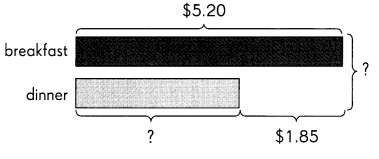Step 2:
Find _____________
Add / Subtract $__________ from$__________.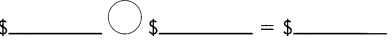Step 3:
Find _____________
Add / Subtract $__________ to$__________.She spends $__________ on both meals. Answer: Step 1: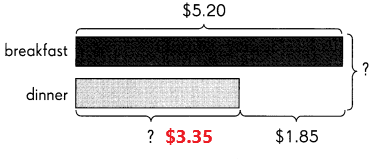Step 2: Find how much she spends on dinner? Subtract$1.85 from $5.20.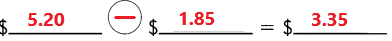Step 3: Find how much she spends on both meals? Add$5.20 to $3.35.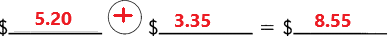She spends$8.55 on both meals.

Look at the problem. Then find the mistake.

Aaron made a mistake while subtracting.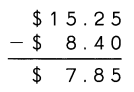Question 2.
Was the mistake made in subtracting the cents?
No, the mistake not made in subtracting the cents.

Question 3.
Was the mistake made in subtracting the dollars?
Yes, the mistake made in subtracting the dollars

Question 4.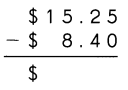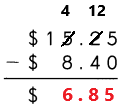Explanation:
Subtract $8.40 from$15.25 the difference is $6.85. The correct answer is$6.85.

Here’s another problem with a mistake.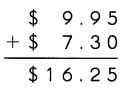Question 5.
What is the mistake?

Question 6.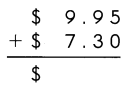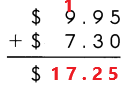Add $9.95 with$7.30 the sum is $17.25. The correct answer is$17.25.

Challenging Practice

Look at the pictures. Solve the real-world problems.

Question 1.
Ms. O’Brien needs to get 6 bottles of shampoo for her childcare center. How much will she spend?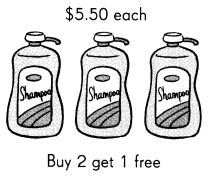For 3 bottles
$5.50 x 2 =$11
For 6 bottles
$11 x 2 =$22
She spend $22. Explanation: Ms. O’Brien needs to get 6 bottles of shampoo for her childcare center. In the above image we can observe Buy 2 get 1 free with a cost of$5.50. Multiply $5.50 with 2 the product is$11. The 3 bottles cost is $11. Multiply$11 with 2 the product is $22. The cost of 6 bottles is$22. She spend $22. Question 2. Mrs. Keith wants to spend the least amount of money to buy 3 gallons of milk. Which bottle should she buy?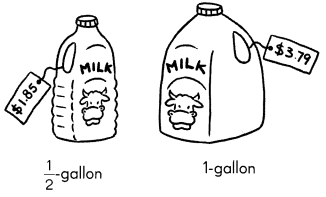Answer: For 1/2 gallon bottle 2 x 1/2 gallon 2 x$1.85 = $3.70 For 1 gallon bottle cost is$3.79.
She buys 1/2 gallon of bottle.
Explanation:
Mrs. Keith wants to spend the least amount of money to buy 3 gallons of milk. The cost of 1/2 gallon bottle is $3.70. The cost of 1 gallon bottle is$3.79. So she buys 1/2 gallon of bottle.

Solve.

Question 1.
Harry has some money. His sister has $75.10. After he gives$28 to his mother, he has $15.20 less than his sister. How much does Harry have at first? Answer:$28 – $15.20 =$12.80
$75.10 +$12.80 = $87.90 Harry have$87.90 at first.
Explanation:
Harry has some money. His sister has $75.10. After he gives$28 to his mother, he has $15.20 less than his sister. Subtract$15.20 from $28 the difference is$12.80.
Add $75.10 with$12.80 the sum is $87.90. Harry have$87.90 at first.

Question 2.
Kate saves some money. She saves only dimes and quarters. She has the same number of dimes and quarters. There are less than 1 2 coins but more than 5 coins altogether. How much could she have saved?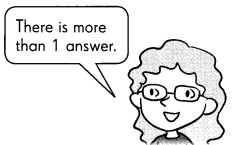$1.05 She have saved$1.05.
Kate saves some money. She saves only dimes and quarters. She has the same number of dimes and quarters. There are less than 12 coins but more than 5 coins altogether. Both 3 dimes and 3 quarters are equal to 6 coins. Multiply 3 with 10¢  the product is 30¢. Multiply 3 with 25¢  the product is 75¢. Add 30¢ with 75¢ the sum is $1.05. She have saved$1.05.